Скачать презентацию Chapter 8 The Complete Response of RL and

4df4cf7faae3148d7e63062a2db8aefb.ppt

• Количество слайдов: 59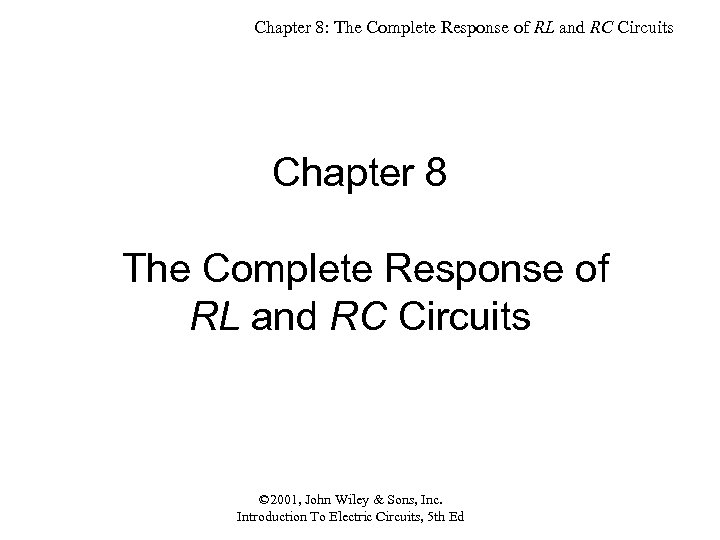Chapter 8: The Complete Response of RL and RC Circuits Chapter 8 The Complete Response of RL and RC Circuits © 2001, John Wiley & Sons, Inc. Introduction To Electric Circuits, 5 th Ed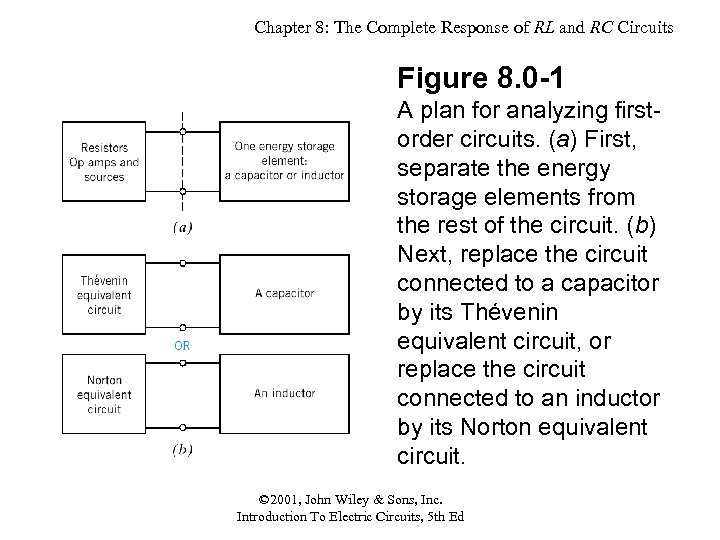Chapter 8: The Complete Response of RL and RC Circuits Figure 8. 0 -1 A plan for analyzing firstorder circuits. (a) First, separate the energy storage elements from the rest of the circuit. (b) Next, replace the circuit connected to a capacitor by its Thévenin equivalent circuit, or replace the circuit connected to an inductor by its Norton equivalent circuit. © 2001, John Wiley & Sons, Inc. Introduction To Electric Circuits, 5 th Ed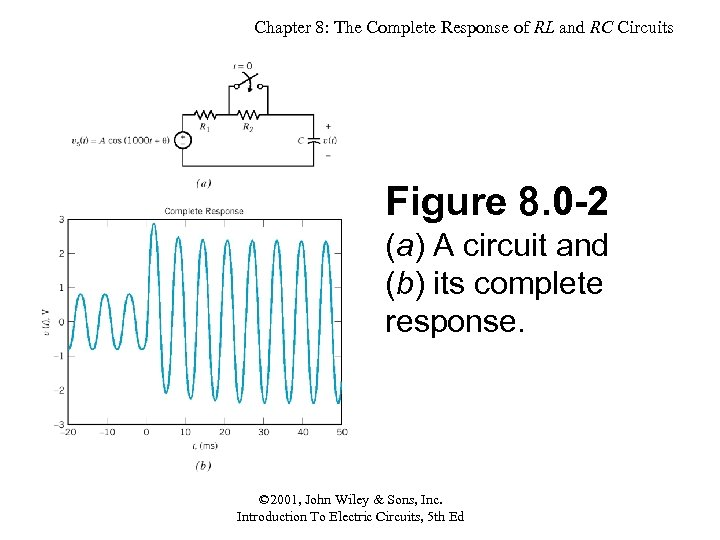Chapter 8: The Complete Response of RL and RC Circuits Figure 8. 0 -2 (a) A circuit and (b) its complete response. © 2001, John Wiley & Sons, Inc. Introduction To Electric Circuits, 5 th Ed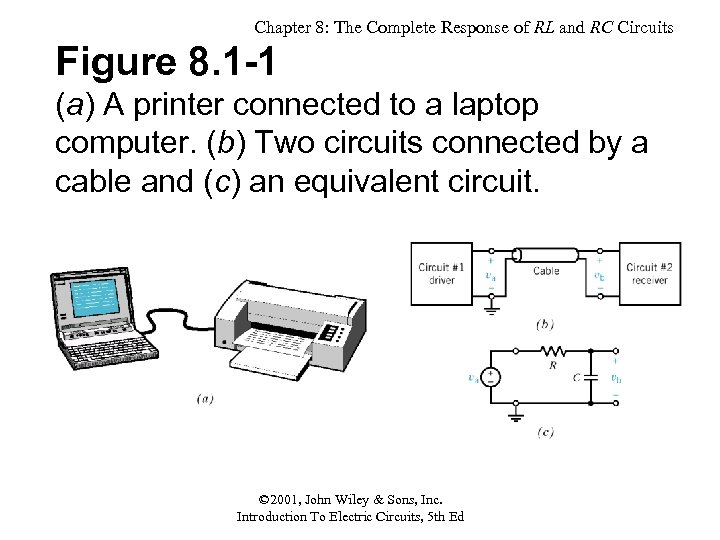Chapter 8: The Complete Response of RL and RC Circuits Figure 8. 1 -1 (a) A printer connected to a laptop computer. (b) Two circuits connected by a cable and (c) an equivalent circuit. © 2001, John Wiley & Sons, Inc. Introduction To Electric Circuits, 5 th Ed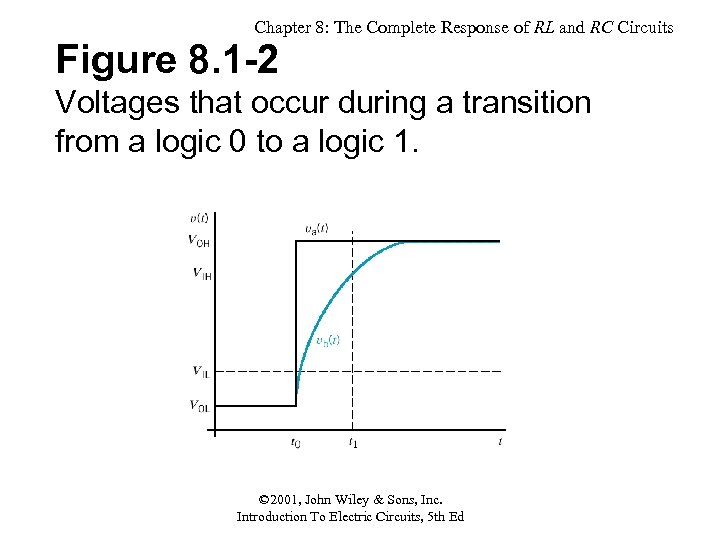Chapter 8: The Complete Response of RL and RC Circuits Figure 8. 1 -2 Voltages that occur during a transition from a logic 0 to a logic 1. © 2001, John Wiley & Sons, Inc. Introduction To Electric Circuits, 5 th Ed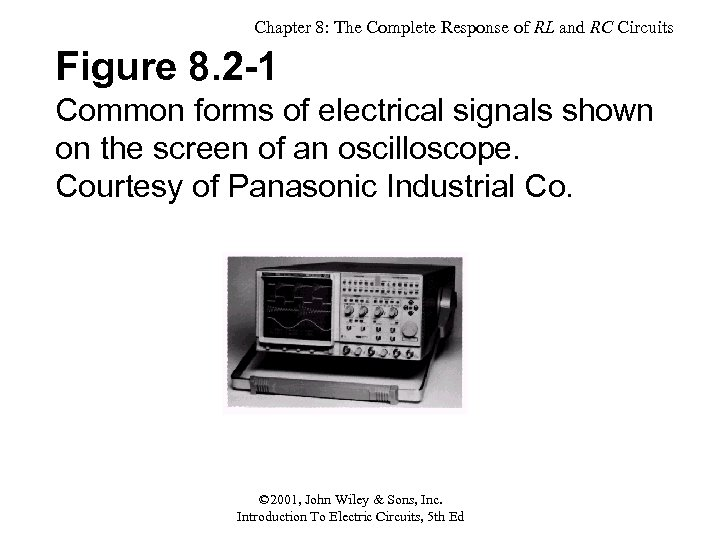Chapter 8: The Complete Response of RL and RC Circuits Figure 8. 2 -1 Common forms of electrical signals shown on the screen of an oscilloscope. Courtesy of Panasonic Industrial Co. © 2001, John Wiley & Sons, Inc. Introduction To Electric Circuits, 5 th Ed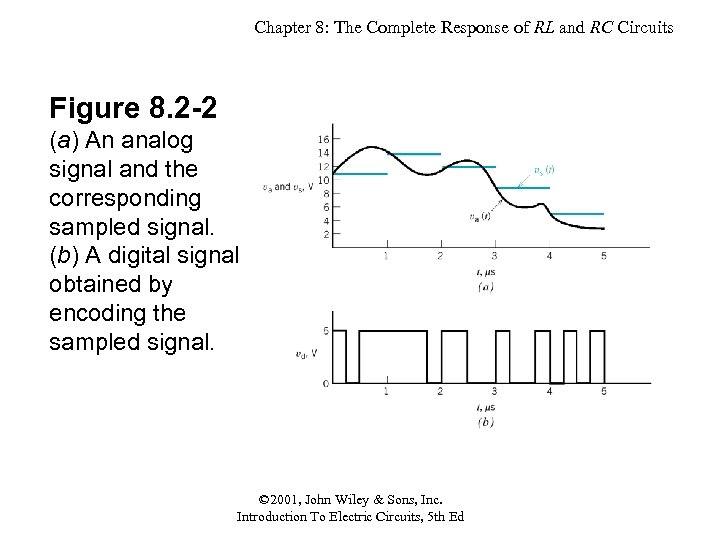Chapter 8: The Complete Response of RL and RC Circuits Figure 8. 2 -2 (a) An analog signal and the corresponding sampled signal. (b) A digital signal obtained by encoding the sampled signal. © 2001, John Wiley & Sons, Inc. Introduction To Electric Circuits, 5 th Ed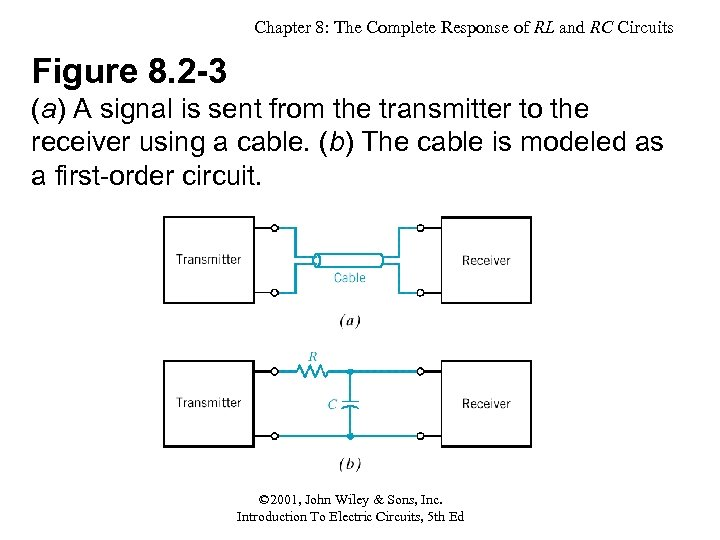Chapter 8: The Complete Response of RL and RC Circuits Figure 8. 2 -3 (a) A signal is sent from the transmitter to the receiver using a cable. (b) The cable is modeled as a first-order circuit. © 2001, John Wiley & Sons, Inc. Introduction To Electric Circuits, 5 th Ed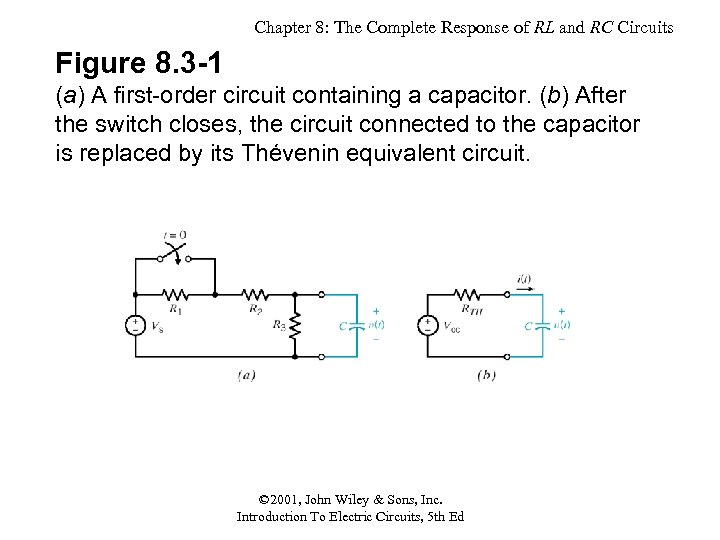Chapter 8: The Complete Response of RL and RC Circuits Figure 8. 3 -1 (a) A first-order circuit containing a capacitor. (b) After the switch closes, the circuit connected to the capacitor is replaced by its Thévenin equivalent circuit. © 2001, John Wiley & Sons, Inc. Introduction To Electric Circuits, 5 th Ed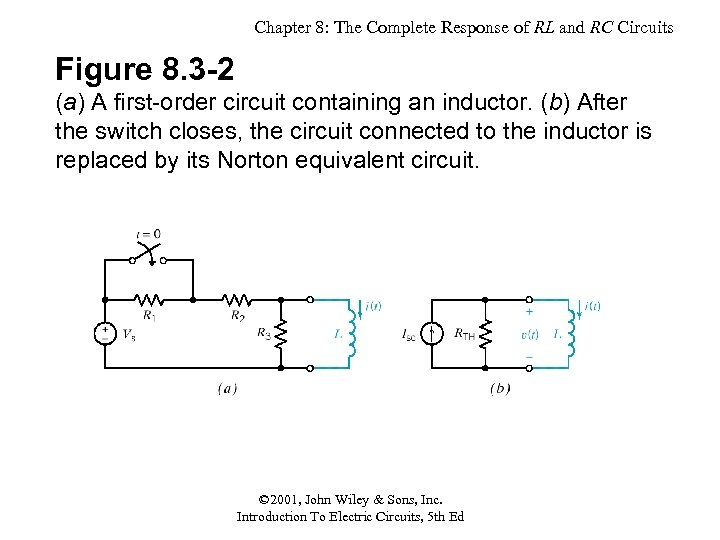Chapter 8: The Complete Response of RL and RC Circuits Figure 8. 3 -2 (a) A first-order circuit containing an inductor. (b) After the switch closes, the circuit connected to the inductor is replaced by its Norton equivalent circuit. © 2001, John Wiley & Sons, Inc. Introduction To Electric Circuits, 5 th Ed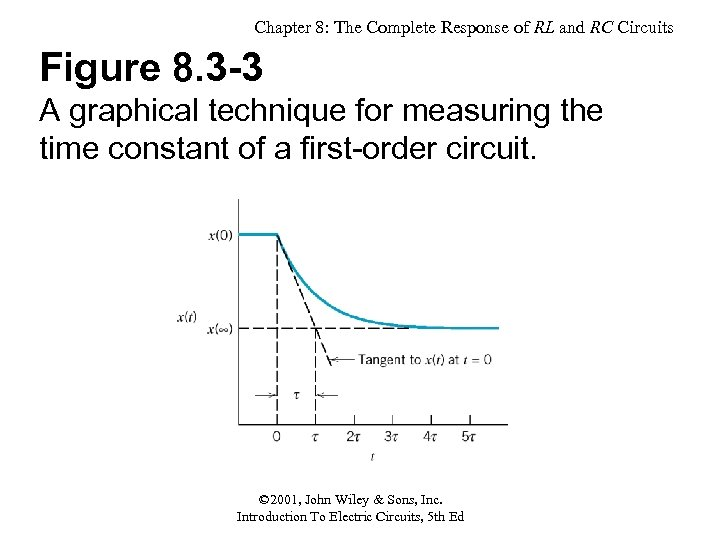Chapter 8: The Complete Response of RL and RC Circuits Figure 8. 3 -3 A graphical technique for measuring the time constant of a first-order circuit. © 2001, John Wiley & Sons, Inc. Introduction To Electric Circuits, 5 th Ed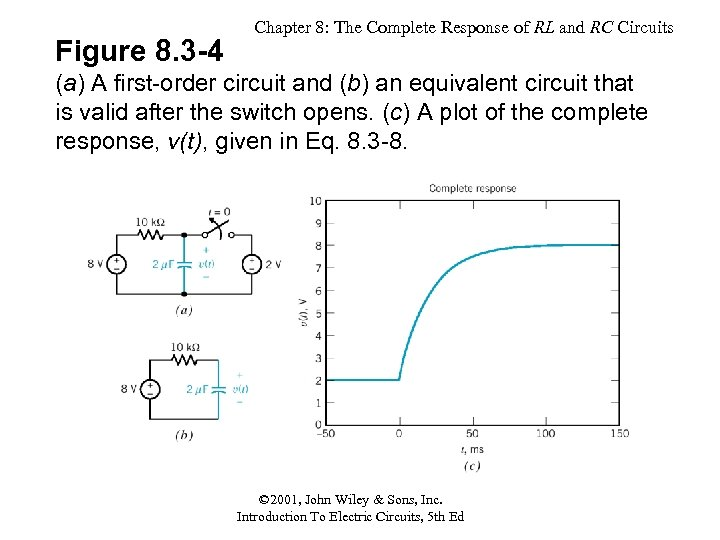Figure 8. 3 -4 Chapter 8: The Complete Response of RL and RC Circuits (a) A first-order circuit and (b) an equivalent circuit that is valid after the switch opens. (c) A plot of the complete response, v(t), given in Eq. 8. 3 -8. © 2001, John Wiley & Sons, Inc. Introduction To Electric Circuits, 5 th Ed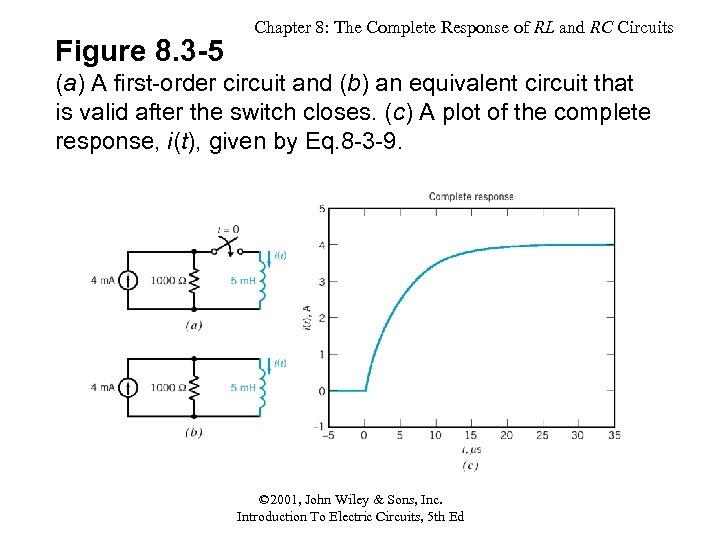Figure 8. 3 -5 Chapter 8: The Complete Response of RL and RC Circuits (a) A first-order circuit and (b) an equivalent circuit that is valid after the switch closes. (c) A plot of the complete response, i(t), given by Eq. 8 -3 -9. © 2001, John Wiley & Sons, Inc. Introduction To Electric Circuits, 5 th Ed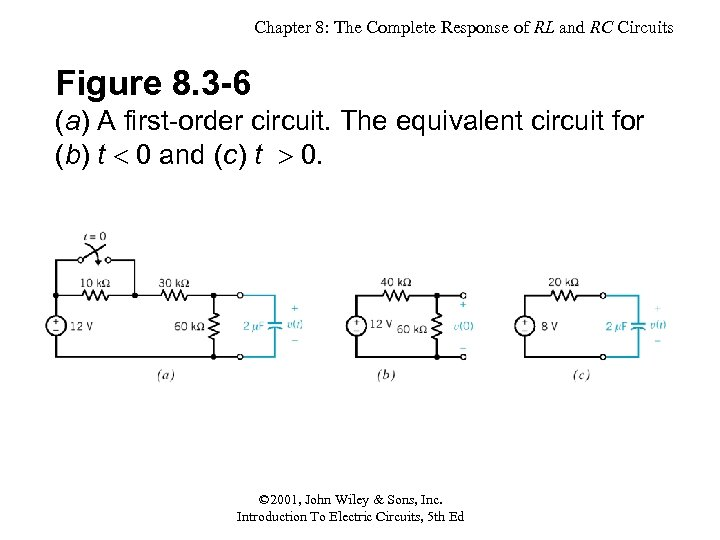Chapter 8: The Complete Response of RL and RC Circuits Figure 8. 3 -6 (a) A first-order circuit. The equivalent circuit for (b) t 0 and (c) t 0. © 2001, John Wiley & Sons, Inc. Introduction To Electric Circuits, 5 th Ed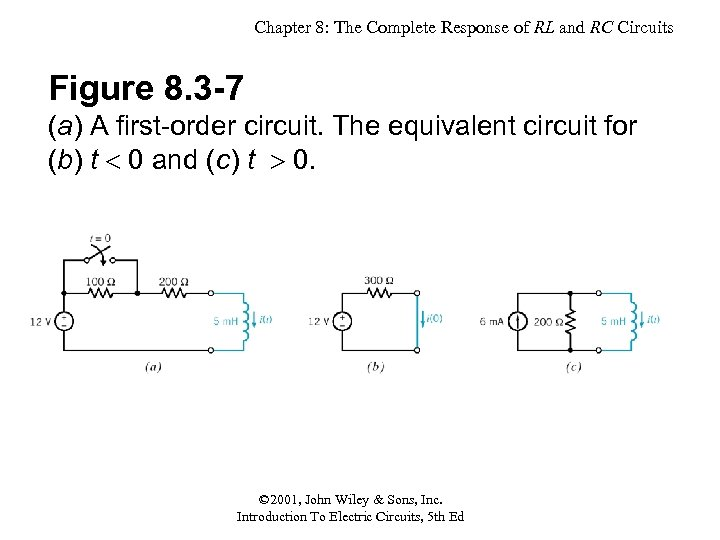Chapter 8: The Complete Response of RL and RC Circuits Figure 8. 3 -7 (a) A first-order circuit. The equivalent circuit for (b) t 0 and (c) t 0. © 2001, John Wiley & Sons, Inc. Introduction To Electric Circuits, 5 th Ed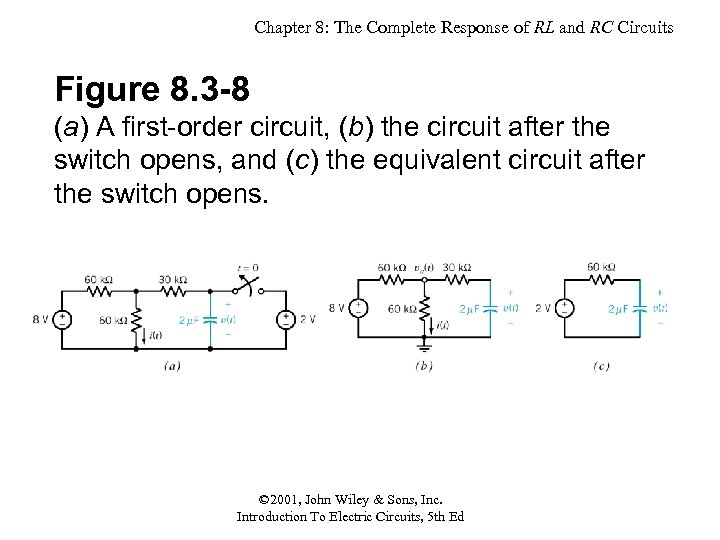Chapter 8: The Complete Response of RL and RC Circuits Figure 8. 3 -8 (a) A first-order circuit, (b) the circuit after the switch opens, and (c) the equivalent circuit after the switch opens. © 2001, John Wiley & Sons, Inc. Introduction To Electric Circuits, 5 th Ed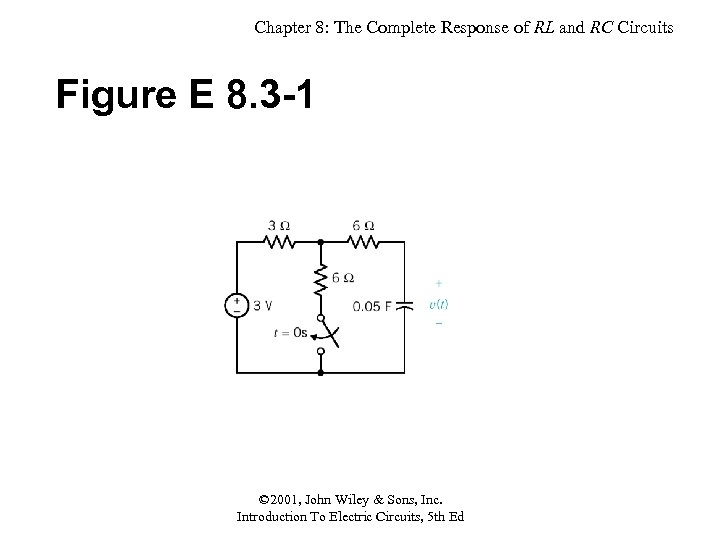Chapter 8: The Complete Response of RL and RC Circuits Figure E 8. 3 -1 © 2001, John Wiley & Sons, Inc. Introduction To Electric Circuits, 5 th Ed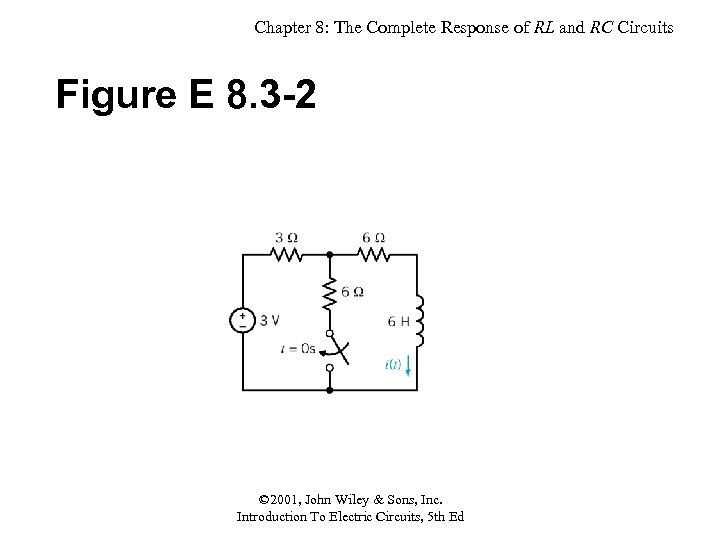Chapter 8: The Complete Response of RL and RC Circuits Figure E 8. 3 -2 © 2001, John Wiley & Sons, Inc. Introduction To Electric Circuits, 5 th Ed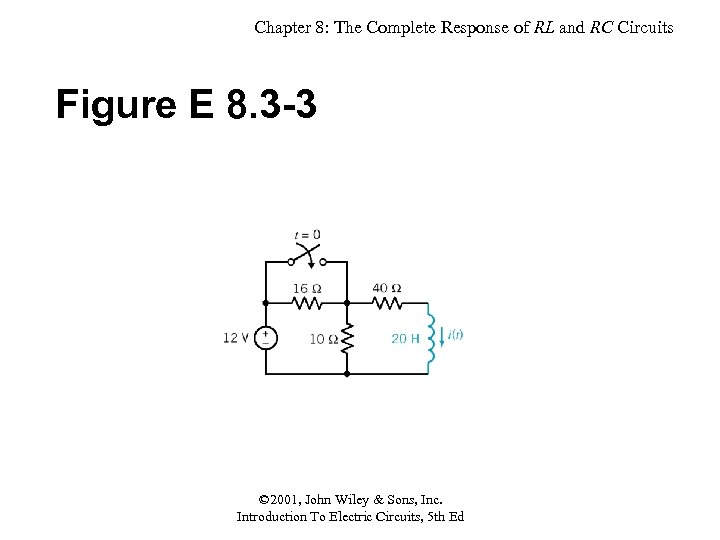Chapter 8: The Complete Response of RL and RC Circuits Figure E 8. 3 -3 © 2001, John Wiley & Sons, Inc. Introduction To Electric Circuits, 5 th Ed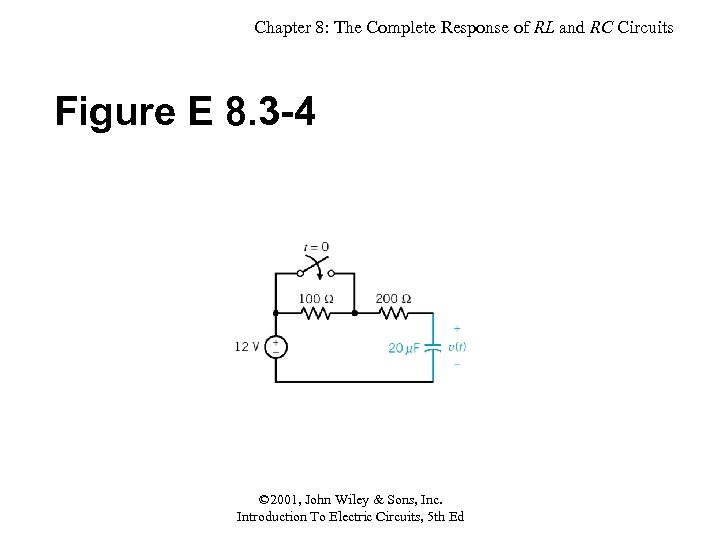Chapter 8: The Complete Response of RL and RC Circuits Figure E 8. 3 -4 © 2001, John Wiley & Sons, Inc. Introduction To Electric Circuits, 5 th Ed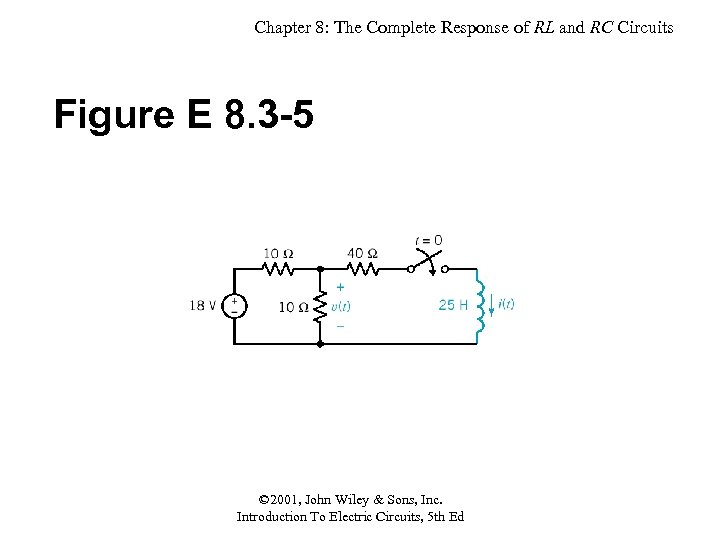Chapter 8: The Complete Response of RL and RC Circuits Figure E 8. 3 -5 © 2001, John Wiley & Sons, Inc. Introduction To Electric Circuits, 5 th Ed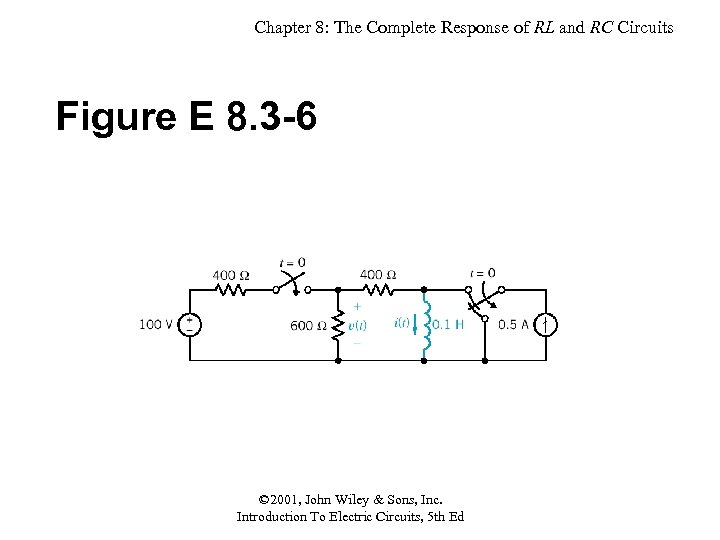Chapter 8: The Complete Response of RL and RC Circuits Figure E 8. 3 -6 © 2001, John Wiley & Sons, Inc. Introduction To Electric Circuits, 5 th Ed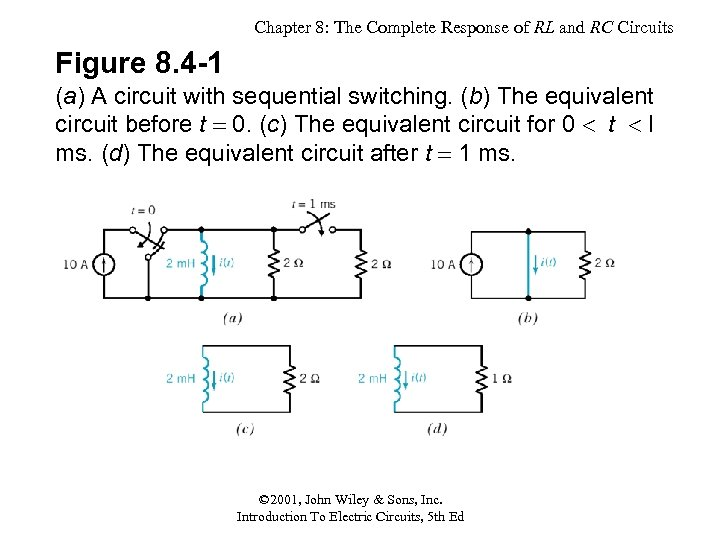Chapter 8: The Complete Response of RL and RC Circuits Figure 8. 4 -1 (a) A circuit with sequential switching. (b) The equivalent circuit before t 0. (c) The equivalent circuit for 0 t l ms. (d) The equivalent circuit after t 1 ms. © 2001, John Wiley & Sons, Inc. Introduction To Electric Circuits, 5 th Ed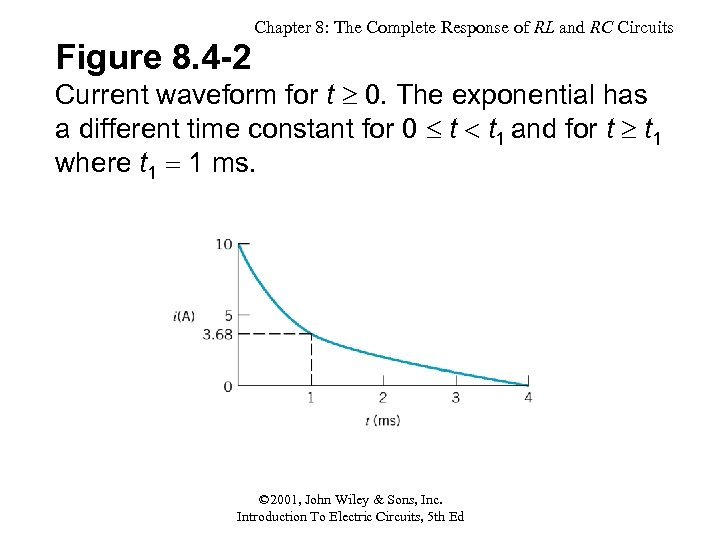Chapter 8: The Complete Response of RL and RC Circuits Figure 8. 4 -2 Current waveform for t 0. The exponential has a different time constant for 0 t t 1 and for t t 1 where t 1 1 ms. © 2001, John Wiley & Sons, Inc. Introduction To Electric Circuits, 5 th Ed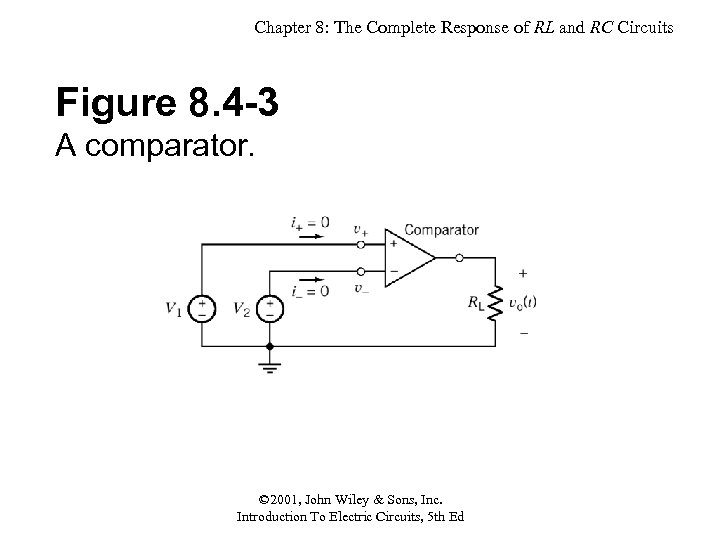Chapter 8: The Complete Response of RL and RC Circuits Figure 8. 4 -3 A comparator. © 2001, John Wiley & Sons, Inc. Introduction To Electric Circuits, 5 th Ed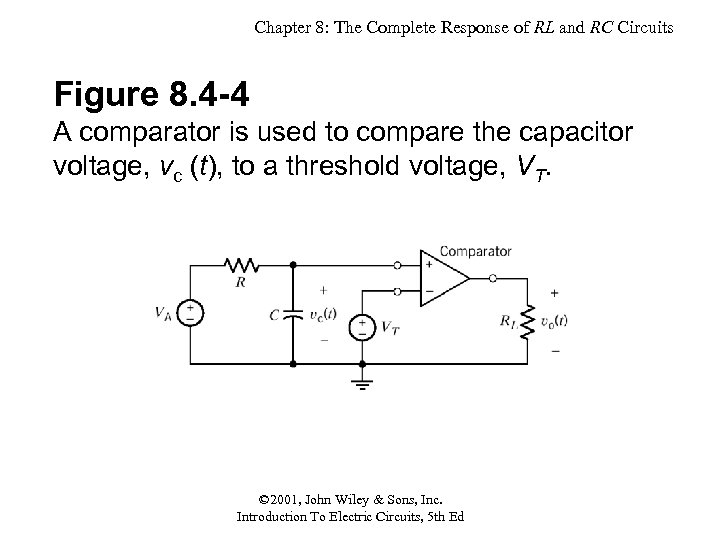Chapter 8: The Complete Response of RL and RC Circuits Figure 8. 4 -4 A comparator is used to compare the capacitor voltage, vc (t), to a threshold voltage, VT. © 2001, John Wiley & Sons, Inc. Introduction To Electric Circuits, 5 th Ed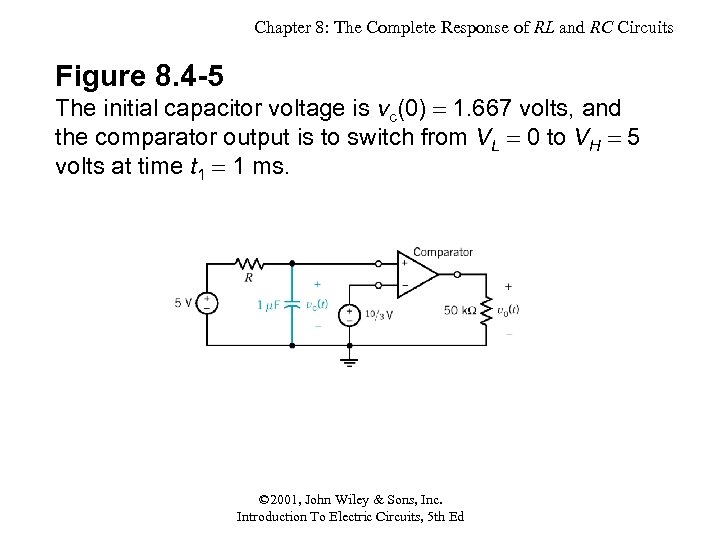Chapter 8: The Complete Response of RL and RC Circuits Figure 8. 4 -5 The initial capacitor voltage is vc(0) 1. 667 volts, and the comparator output is to switch from VL 0 to VH 5 volts at time t 1 1 ms. © 2001, John Wiley & Sons, Inc. Introduction To Electric Circuits, 5 th Ed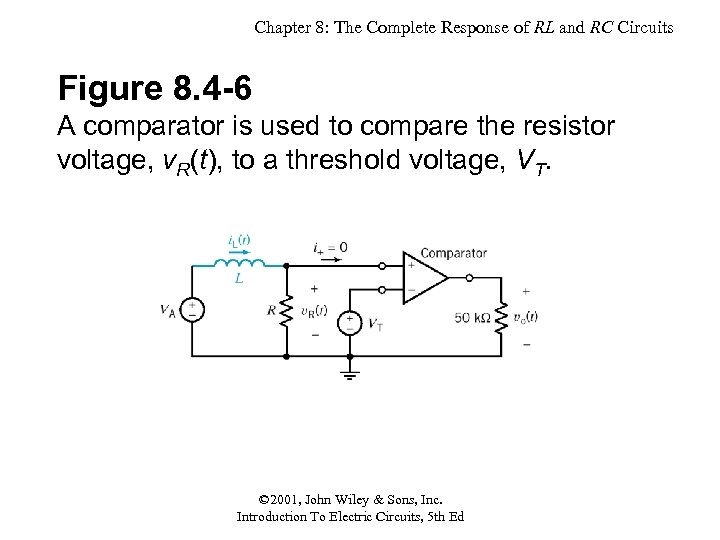Chapter 8: The Complete Response of RL and RC Circuits Figure 8. 4 -6 A comparator is used to compare the resistor voltage, v. R(t), to a threshold voltage, VT. © 2001, John Wiley & Sons, Inc. Introduction To Electric Circuits, 5 th Ed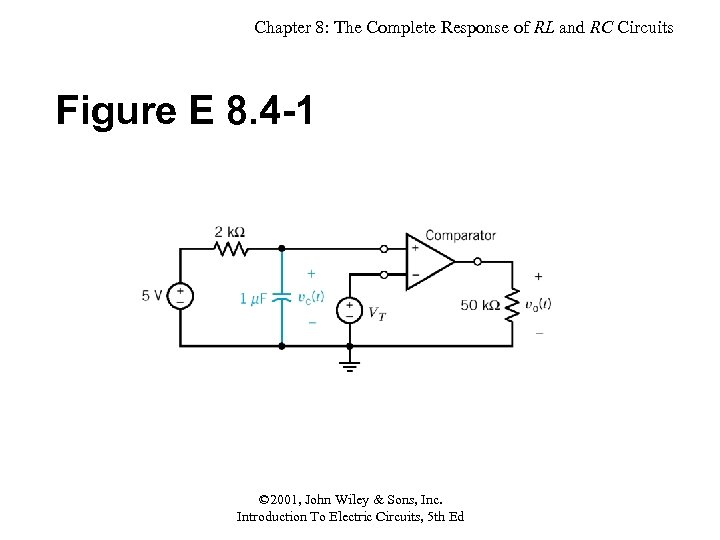Chapter 8: The Complete Response of RL and RC Circuits Figure E 8. 4 -1 © 2001, John Wiley & Sons, Inc. Introduction To Electric Circuits, 5 th Ed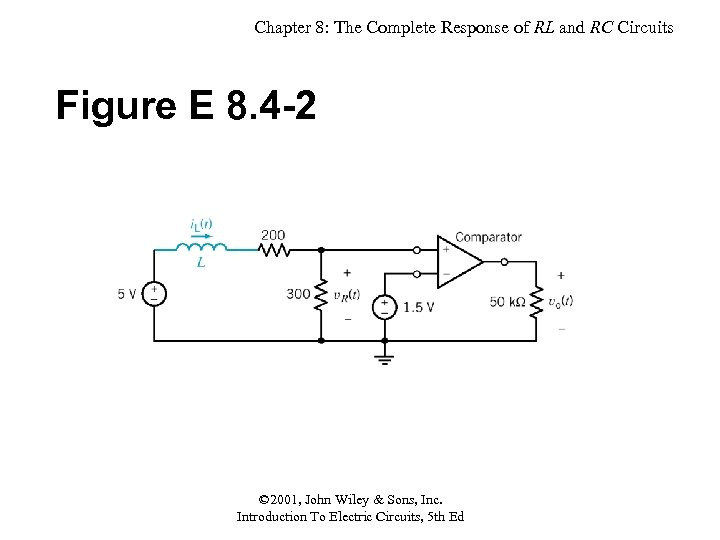Chapter 8: The Complete Response of RL and RC Circuits Figure E 8. 4 -2 © 2001, John Wiley & Sons, Inc. Introduction To Electric Circuits, 5 th Ed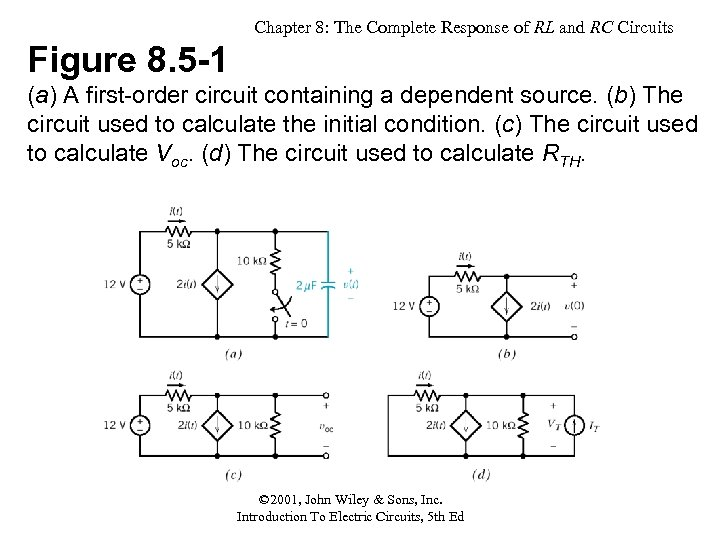Chapter 8: The Complete Response of RL and RC Circuits Figure 8. 5 -1 (a) A first-order circuit containing a dependent source. (b) The circuit used to calculate the initial condition. (c) The circuit used to calculate Voc. (d) The circuit used to calculate RTH. © 2001, John Wiley & Sons, Inc. Introduction To Electric Circuits, 5 th Ed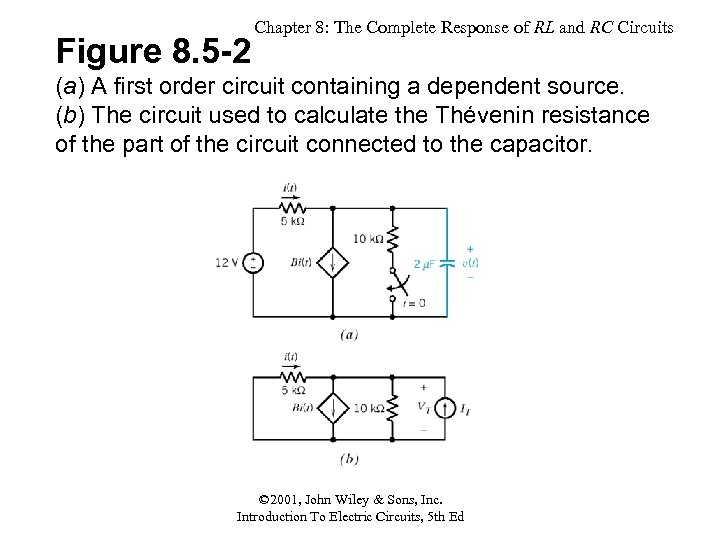Figure 8. 5 -2 Chapter 8: The Complete Response of RL and RC Circuits (a) A first order circuit containing a dependent source. (b) The circuit used to calculate the Thévenin resistance of the part of the circuit connected to the capacitor. © 2001, John Wiley & Sons, Inc. Introduction To Electric Circuits, 5 th Ed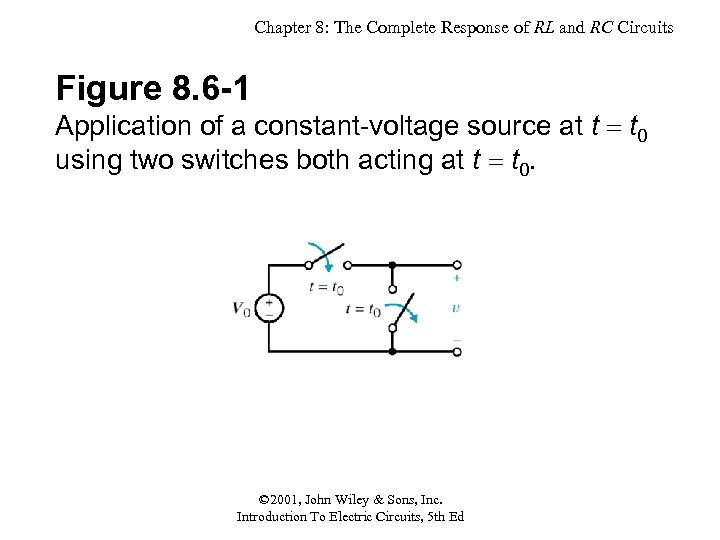Chapter 8: The Complete Response of RL and RC Circuits Figure 8. 6 -1 Application of a constant-voltage source at t t 0 using two switches both acting at t t 0. © 2001, John Wiley & Sons, Inc. Introduction To Electric Circuits, 5 th Ed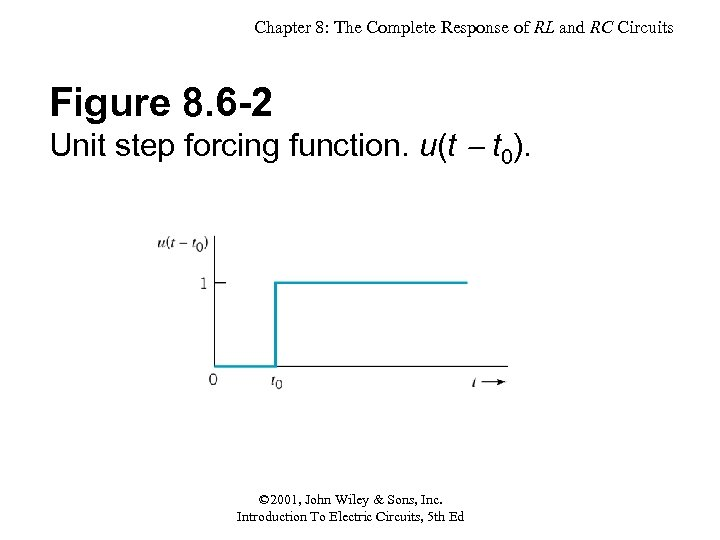Chapter 8: The Complete Response of RL and RC Circuits Figure 8. 6 -2 Unit step forcing function. u(t t 0). © 2001, John Wiley & Sons, Inc. Introduction To Electric Circuits, 5 th Ed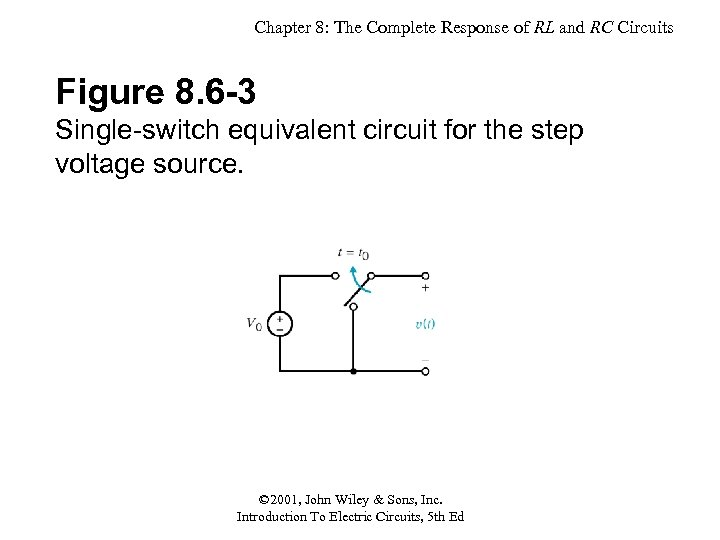Chapter 8: The Complete Response of RL and RC Circuits Figure 8. 6 -3 Single-switch equivalent circuit for the step voltage source. © 2001, John Wiley & Sons, Inc. Introduction To Electric Circuits, 5 th Ed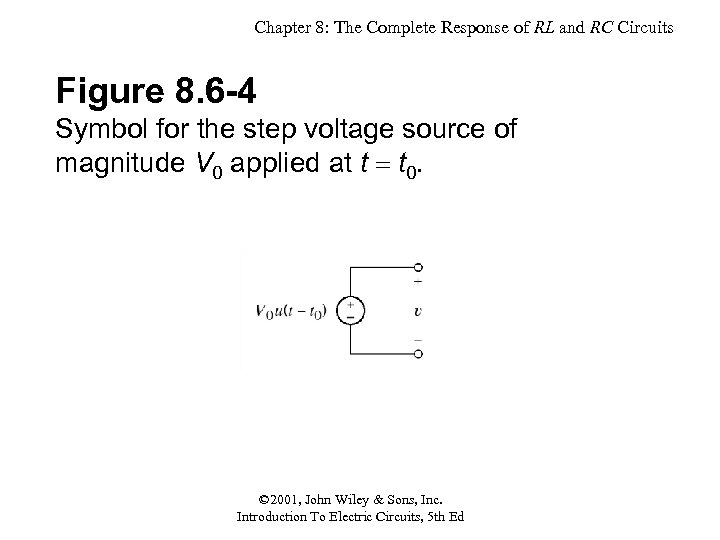Chapter 8: The Complete Response of RL and RC Circuits Figure 8. 6 -4 Symbol for the step voltage source of magnitude V 0 applied at t t 0. © 2001, John Wiley & Sons, Inc. Introduction To Electric Circuits, 5 th Ed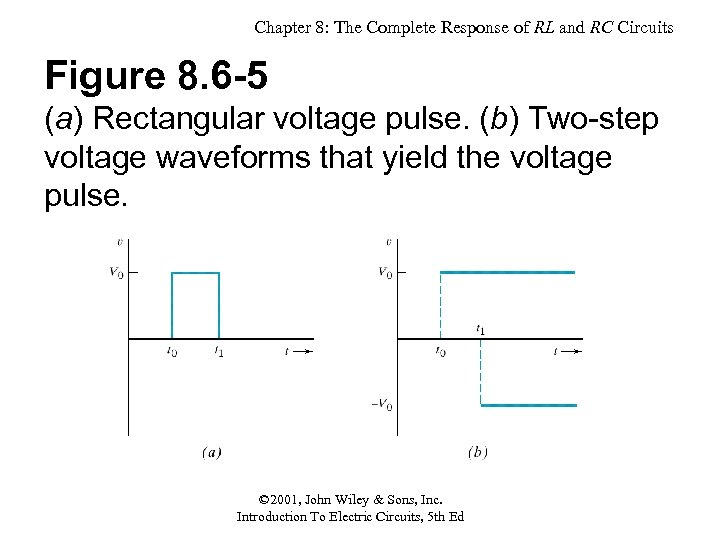Chapter 8: The Complete Response of RL and RC Circuits Figure 8. 6 -5 (a) Rectangular voltage pulse. (b) Two-step voltage waveforms that yield the voltage pulse. © 2001, John Wiley & Sons, Inc. Introduction To Electric Circuits, 5 th Ed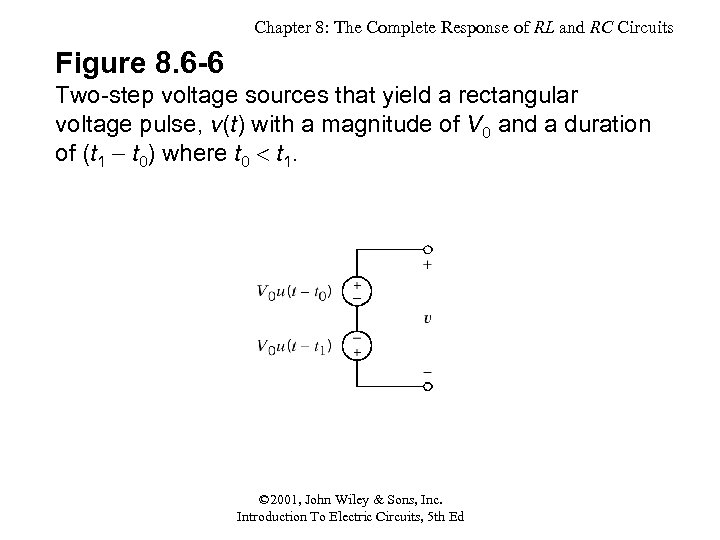Chapter 8: The Complete Response of RL and RC Circuits Figure 8. 6 -6 Two-step voltage sources that yield a rectangular voltage pulse, v(t) with a magnitude of V 0 and a duration of (t 1 t 0) where t 0 t 1. © 2001, John Wiley & Sons, Inc. Introduction To Electric Circuits, 5 th Ed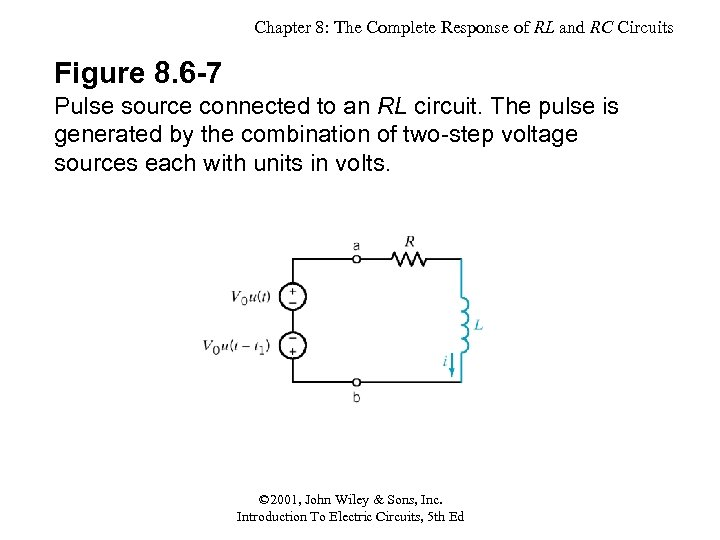Chapter 8: The Complete Response of RL and RC Circuits Figure 8. 6 -7 Pulse source connected to an RL circuit. The pulse is generated by the combination of two-step voltage sources each with units in volts. © 2001, John Wiley & Sons, Inc. Introduction To Electric Circuits, 5 th Ed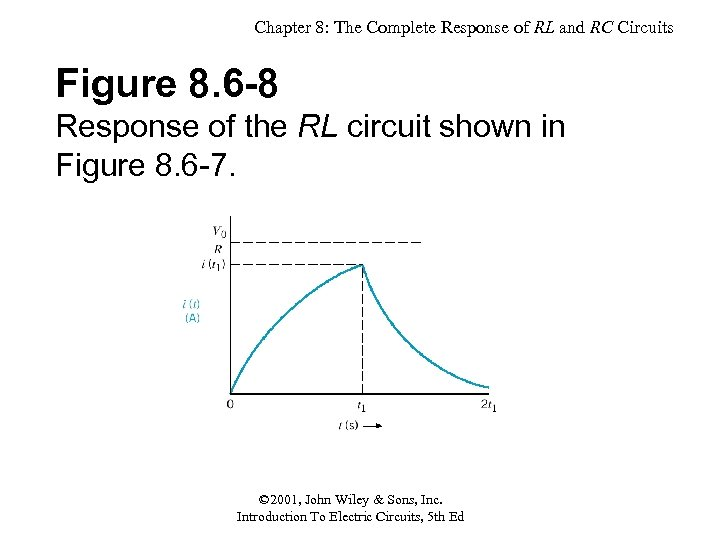Chapter 8: The Complete Response of RL and RC Circuits Figure 8. 6 -8 Response of the RL circuit shown in Figure 8. 6 -7. © 2001, John Wiley & Sons, Inc. Introduction To Electric Circuits, 5 th Ed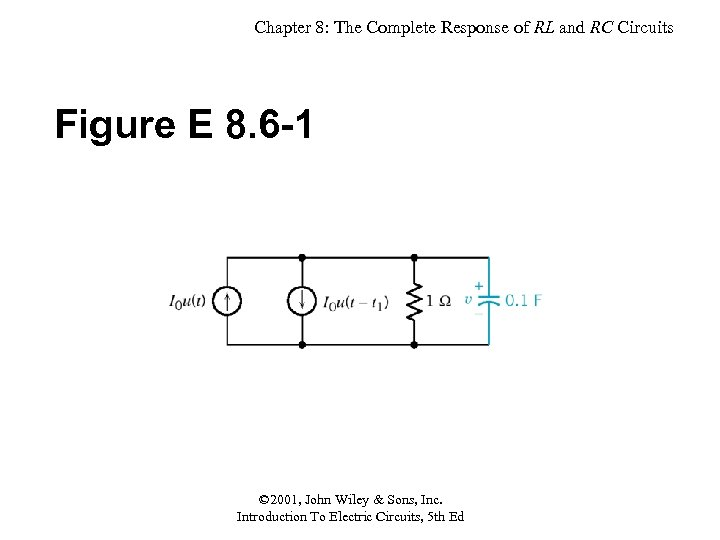Chapter 8: The Complete Response of RL and RC Circuits Figure E 8. 6 -1 © 2001, John Wiley & Sons, Inc. Introduction To Electric Circuits, 5 th Ed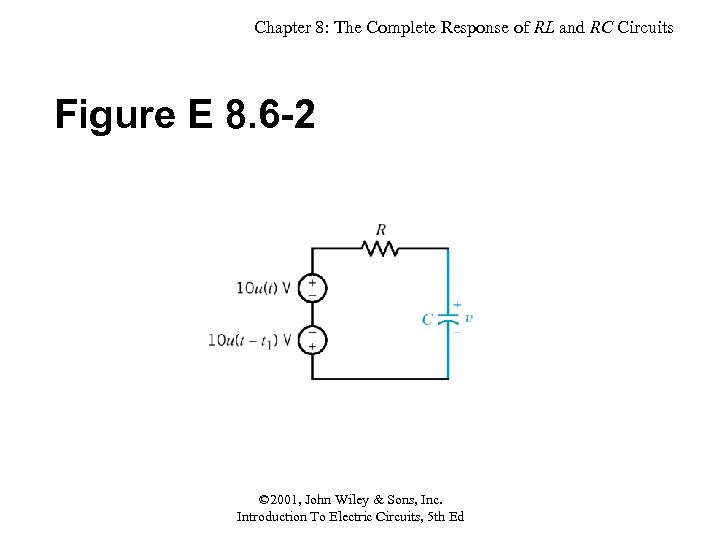Chapter 8: The Complete Response of RL and RC Circuits Figure E 8. 6 -2 © 2001, John Wiley & Sons, Inc. Introduction To Electric Circuits, 5 th Ed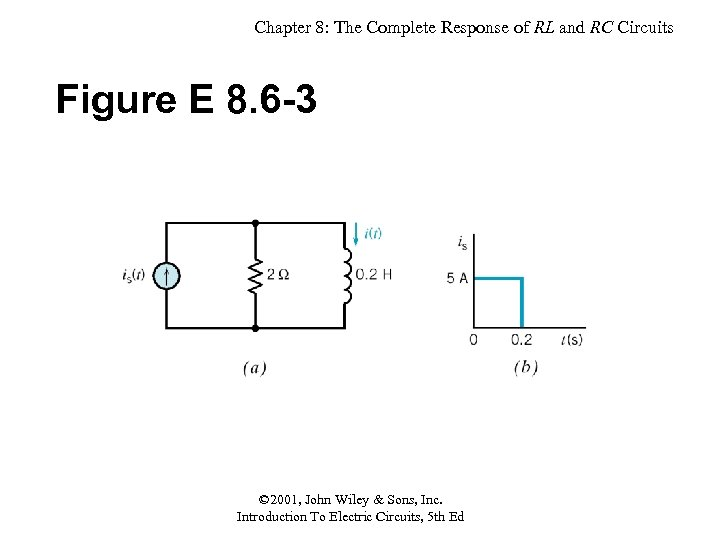Chapter 8: The Complete Response of RL and RC Circuits Figure E 8. 6 -3 © 2001, John Wiley & Sons, Inc. Introduction To Electric Circuits, 5 th Ed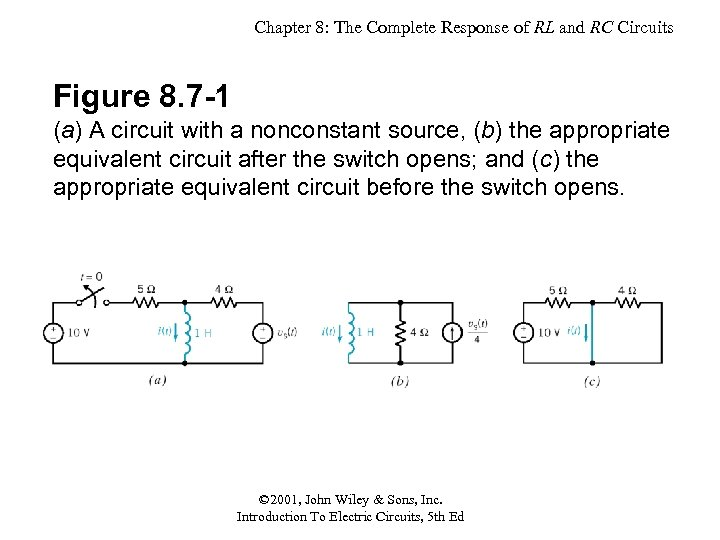Chapter 8: The Complete Response of RL and RC Circuits Figure 8. 7 -1 (a) A circuit with a nonconstant source, (b) the appropriate equivalent circuit after the switch opens; and (c) the appropriate equivalent circuit before the switch opens. © 2001, John Wiley & Sons, Inc. Introduction To Electric Circuits, 5 th Ed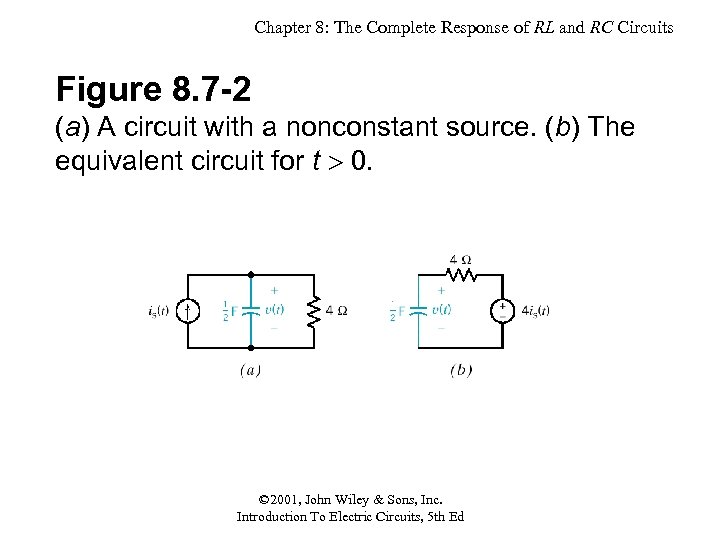Chapter 8: The Complete Response of RL and RC Circuits Figure 8. 7 -2 (a) A circuit with a nonconstant source. (b) The equivalent circuit for t 0. © 2001, John Wiley & Sons, Inc. Introduction To Electric Circuits, 5 th Ed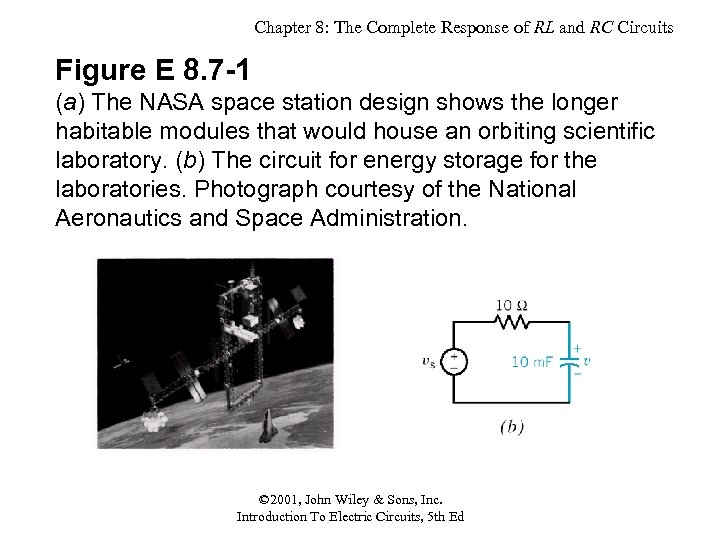Chapter 8: The Complete Response of RL and RC Circuits Figure E 8. 7 -1 (a) The NASA space station design shows the longer habitable modules that would house an orbiting scientific laboratory. (b) The circuit for energy storage for the laboratories. Photograph courtesy of the National Aeronautics and Space Administration. © 2001, John Wiley & Sons, Inc. Introduction To Electric Circuits, 5 th Ed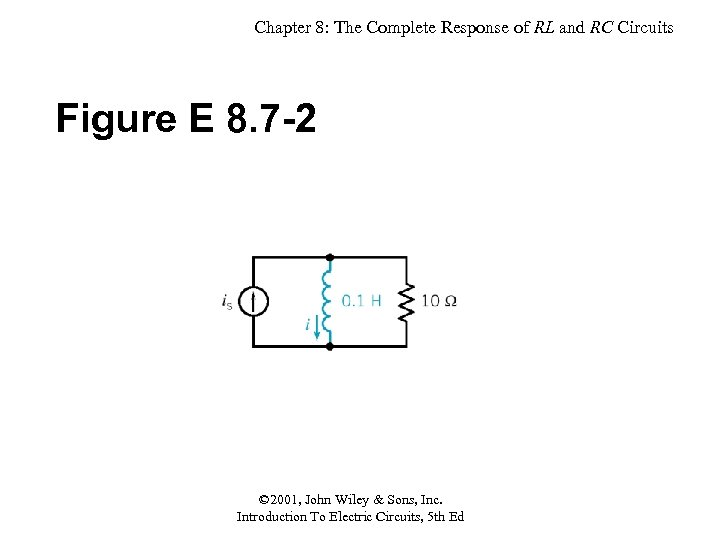Chapter 8: The Complete Response of RL and RC Circuits Figure E 8. 7 -2 © 2001, John Wiley & Sons, Inc. Introduction To Electric Circuits, 5 th Ed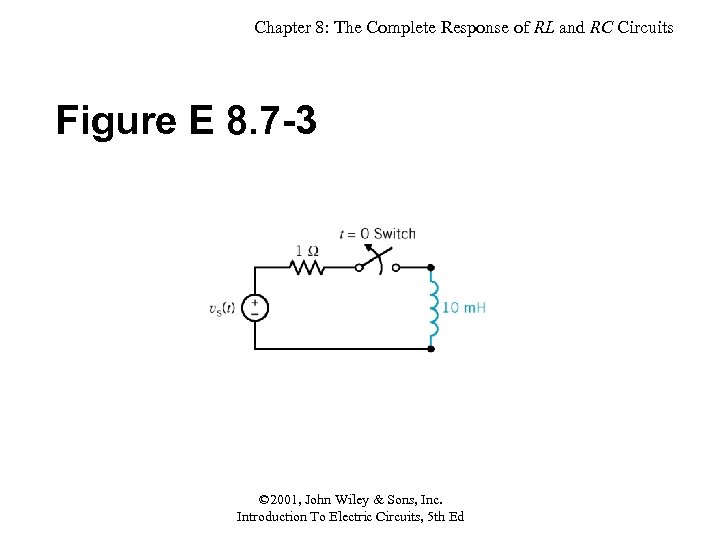Chapter 8: The Complete Response of RL and RC Circuits Figure E 8. 7 -3 © 2001, John Wiley & Sons, Inc. Introduction To Electric Circuits, 5 th Ed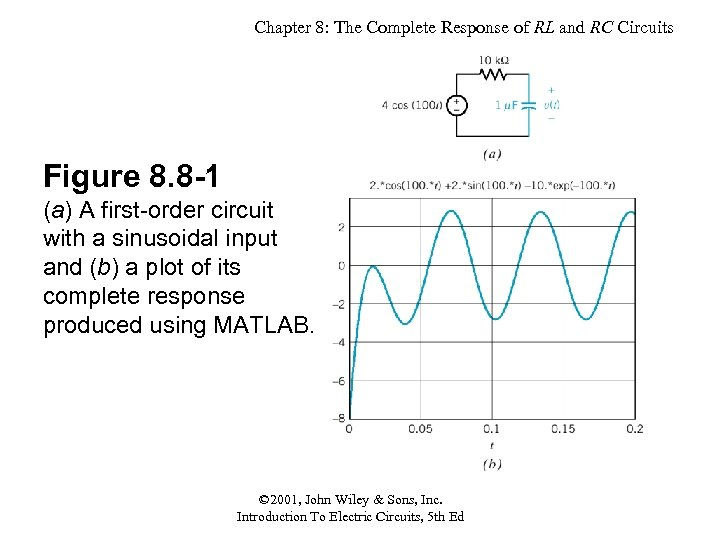Chapter 8: The Complete Response of RL and RC Circuits Figure 8. 8 -1 (a) A first-order circuit with a sinusoidal input and (b) a plot of its complete response produced using MATLAB. © 2001, John Wiley & Sons, Inc. Introduction To Electric Circuits, 5 th Ed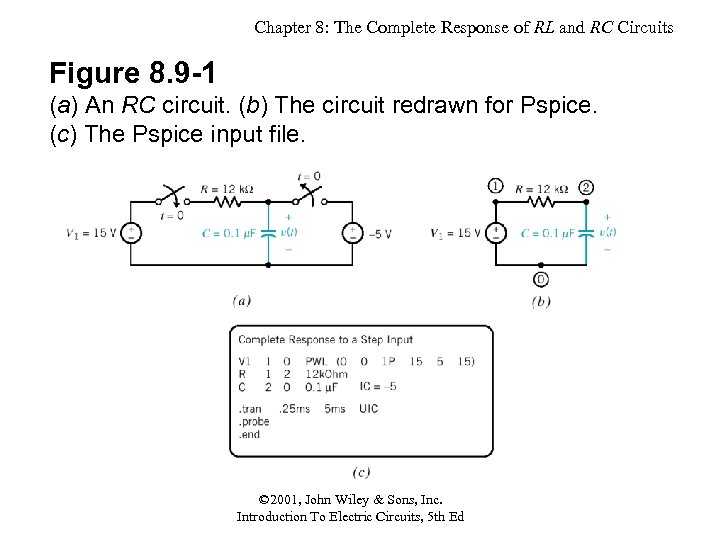Chapter 8: The Complete Response of RL and RC Circuits Figure 8. 9 -1 (a) An RC circuit. (b) The circuit redrawn for Pspice. (c) The Pspice input file. © 2001, John Wiley & Sons, Inc. Introduction To Electric Circuits, 5 th Ed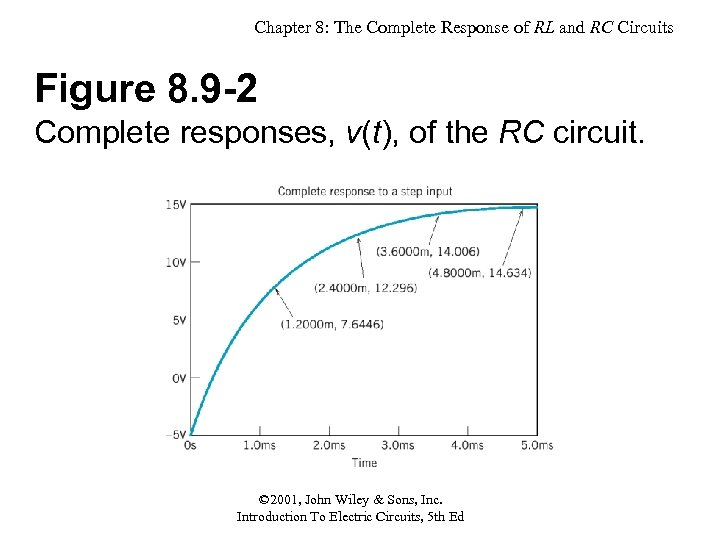Chapter 8: The Complete Response of RL and RC Circuits Figure 8. 9 -2 Complete responses, v(t), of the RC circuit. © 2001, John Wiley & Sons, Inc. Introduction To Electric Circuits, 5 th Ed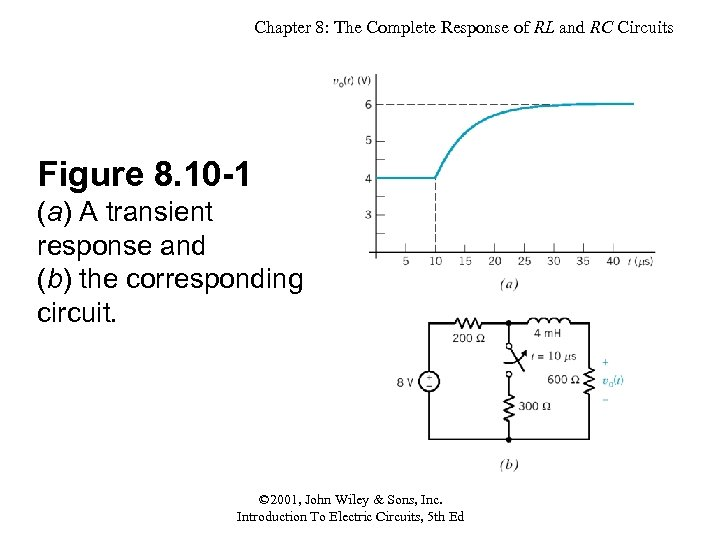Chapter 8: The Complete Response of RL and RC Circuits Figure 8. 10 -1 (a) A transient response and (b) the corresponding circuit. © 2001, John Wiley & Sons, Inc. Introduction To Electric Circuits, 5 th Ed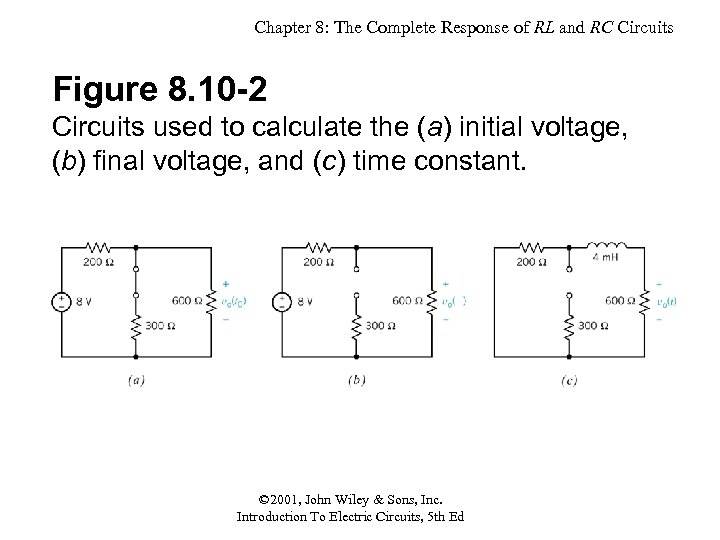Chapter 8: The Complete Response of RL and RC Circuits Figure 8. 10 -2 Circuits used to calculate the (a) initial voltage, (b) final voltage, and (c) time constant. © 2001, John Wiley & Sons, Inc. Introduction To Electric Circuits, 5 th Ed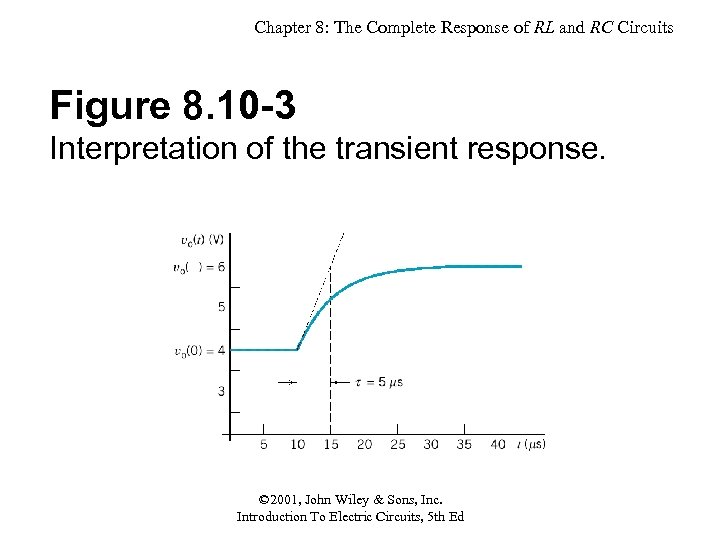Chapter 8: The Complete Response of RL and RC Circuits Figure 8. 10 -3 Interpretation of the transient response. © 2001, John Wiley & Sons, Inc. Introduction To Electric Circuits, 5 th Ed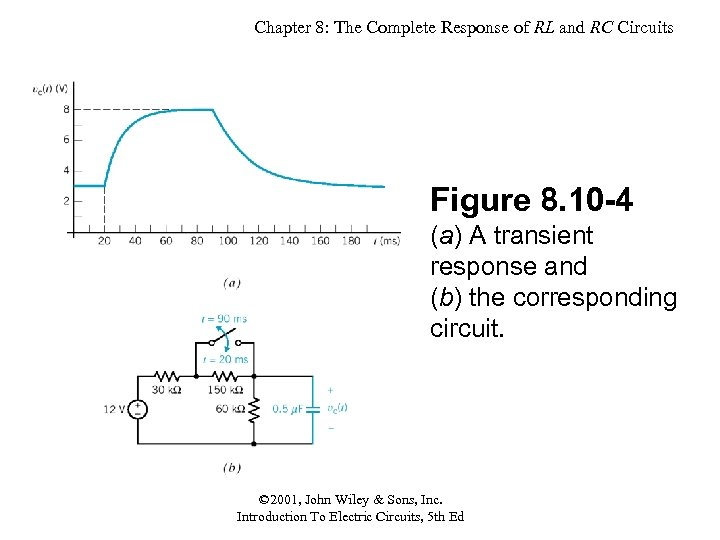Chapter 8: The Complete Response of RL and RC Circuits Figure 8. 10 -4 (a) A transient response and (b) the corresponding circuit. © 2001, John Wiley & Sons, Inc. Introduction To Electric Circuits, 5 th Ed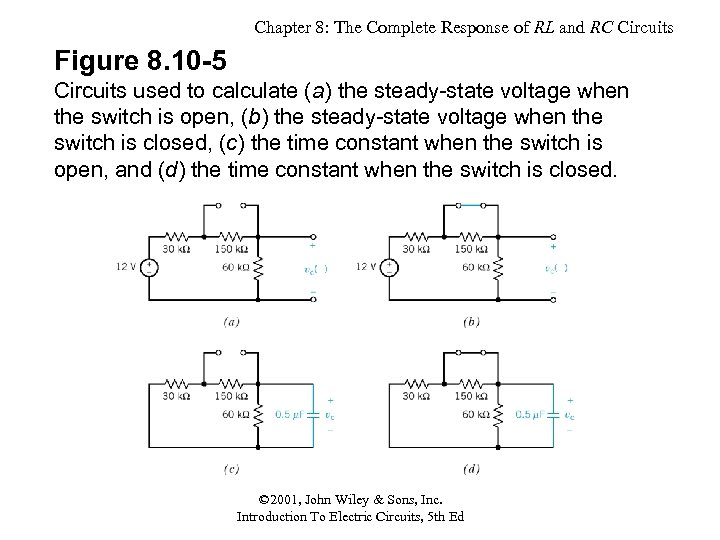Chapter 8: The Complete Response of RL and RC Circuits Figure 8. 10 -5 Circuits used to calculate (a) the steady-state voltage when the switch is open, (b) the steady-state voltage when the switch is closed, (c) the time constant when the switch is open, and (d) the time constant when the switch is closed. © 2001, John Wiley & Sons, Inc. Introduction To Electric Circuits, 5 th Ed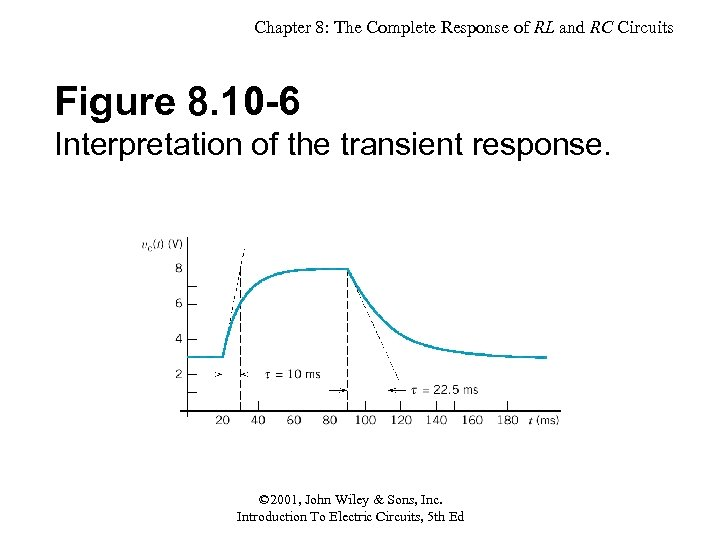Chapter 8: The Complete Response of RL and RC Circuits Figure 8. 10 -6 Interpretation of the transient response. © 2001, John Wiley & Sons, Inc. Introduction To Electric Circuits, 5 th Ed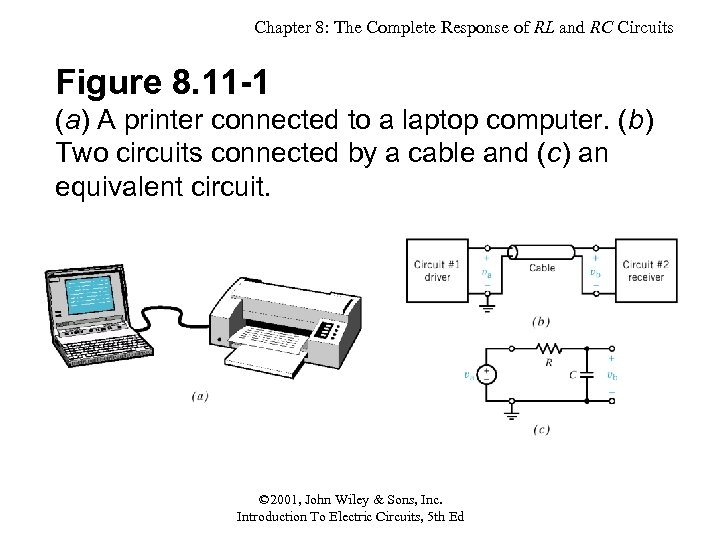Chapter 8: The Complete Response of RL and RC Circuits Figure 8. 11 -1 (a) A printer connected to a laptop computer. (b) Two circuits connected by a cable and (c) an equivalent circuit. © 2001, John Wiley & Sons, Inc. Introduction To Electric Circuits, 5 th Ed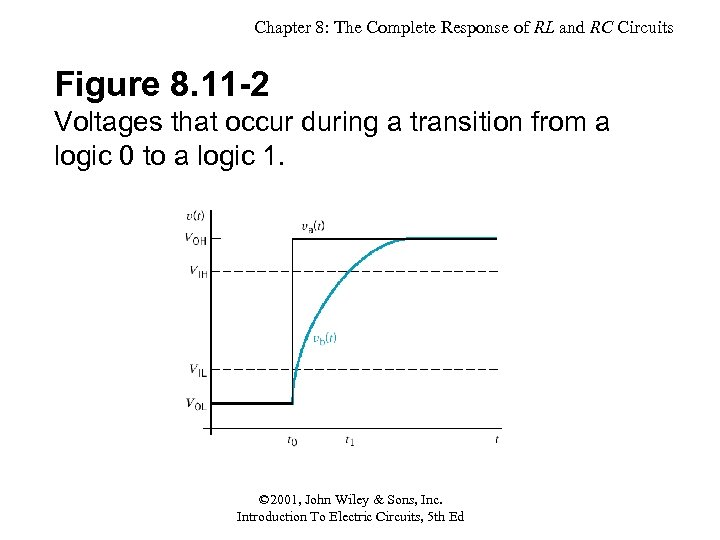Chapter 8: The Complete Response of RL and RC Circuits Figure 8. 11 -2 Voltages that occur during a transition from a logic 0 to a logic 1. © 2001, John Wiley & Sons, Inc. Introduction To Electric Circuits, 5 th Ed